# How to select a subset of a DataFrame?

• Last Updated : 02 Jun, 2021

In this article, we are going to discuss how to select a subset of columns and rows from a DataFrame. We are going to use the nba.csv dataset to perform all operations.

Attention geek! Strengthen your foundations with the Python Programming Foundation Course and learn the basics.

To begin with, your interview preparations Enhance your Data Structures concepts with the Python DS Course. And to begin with your Machine Learning Journey, join the Machine Learning - Basic Level Course

## Python3

 `# import required module``import` `pandas as pd` `# assign dataframe``data ``=` `pd.read_csv(``"nba.csv"``)` `# display dataframe``data.head()`

Output: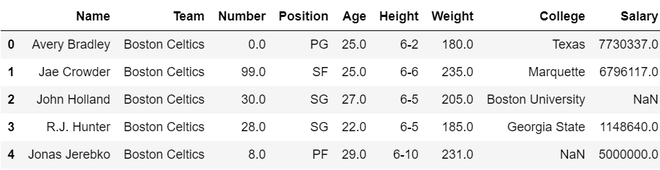Below are various operations by using which we can select a subset for a given dataframe:

• Select a specific column from a dataframe

To select a single column, we can use a square bracket [ ]:

## Python3

 `# import required module``import` `pandas as pd` `# assign dataframe``data ``=` `pd.read_csv(``"nba.csv"``)` `# get a single columns``ages ``=` `data[``"Age"``]` `# display the column``ages.head()`

Output: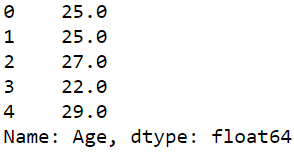• Select multiple columns from a dataframe

We can pass a list of column names inside the square bracket [] to get multiple columns:

## Python3

 `# import required module``import` `pandas as pd` `# assign dataframe``data ``=` `pd.read_csv(``"nba.csv"``)` `# get a single columns``name_sex ``=` `data[[``"Name"``,``"Age"``]]` `# display the column``name_sex.head()`

Output: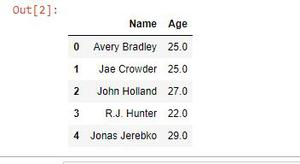• Select a subset of rows from a dataframe

To select rows of people older than 25 years in the given dataset, we can put conditions within the brackets to select specific rows depending on the condition.

## Python3

 `# importing pandas library``import` `pandas as pd` `# reading csv file``data ``=` `pd.read_csv(``"nba.csv"``)` `# subset of dataframe``above_25 ``=` `data[data[``"Age"``] > ``35``]` `# display subset``print``(above_25.head())`

Output: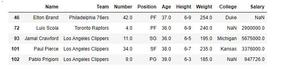• Select a subset of rows and columns combined

In this case, a subset of all rows and columns is made in one go, and select [] is not sufficient now. The loc or iloc operators are needed. The section before the comma is the rows you choose, and the part after the comma is the columns you want to pick by using loc or iloc. Here we select only names of people older than 25.

## Python3

 `# importing pandas library``import` `pandas as pd` `# reading csv file``data ``=` `pd.read_csv(``"nba.csv"``)` `# subset of dataframe``adults ``=` `data.loc[data[``"Age"``] > ``25``, ``"Name"``]` `# display susbset``print``(adults.head())`

Output: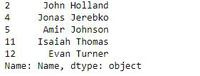My Personal Notes arrow_drop_up